Learn All Concepts of Chapter 1 Class 9 - FREE. Check - Chapter 1 Class 9 Maths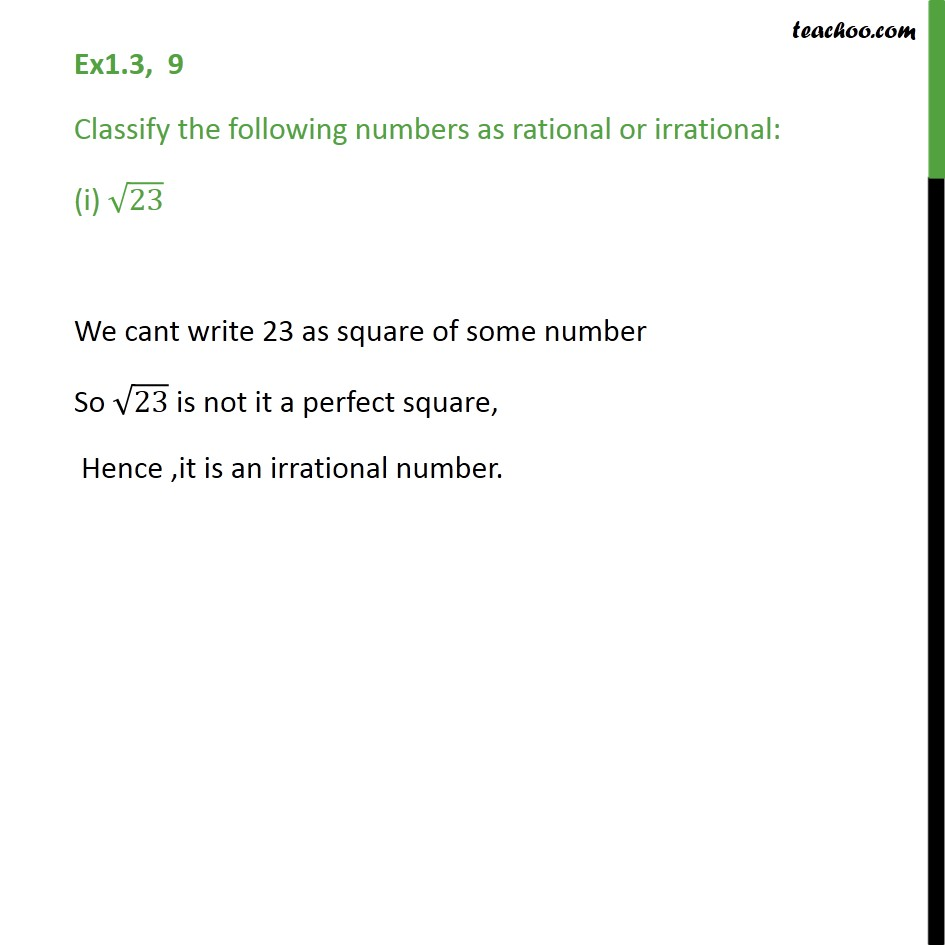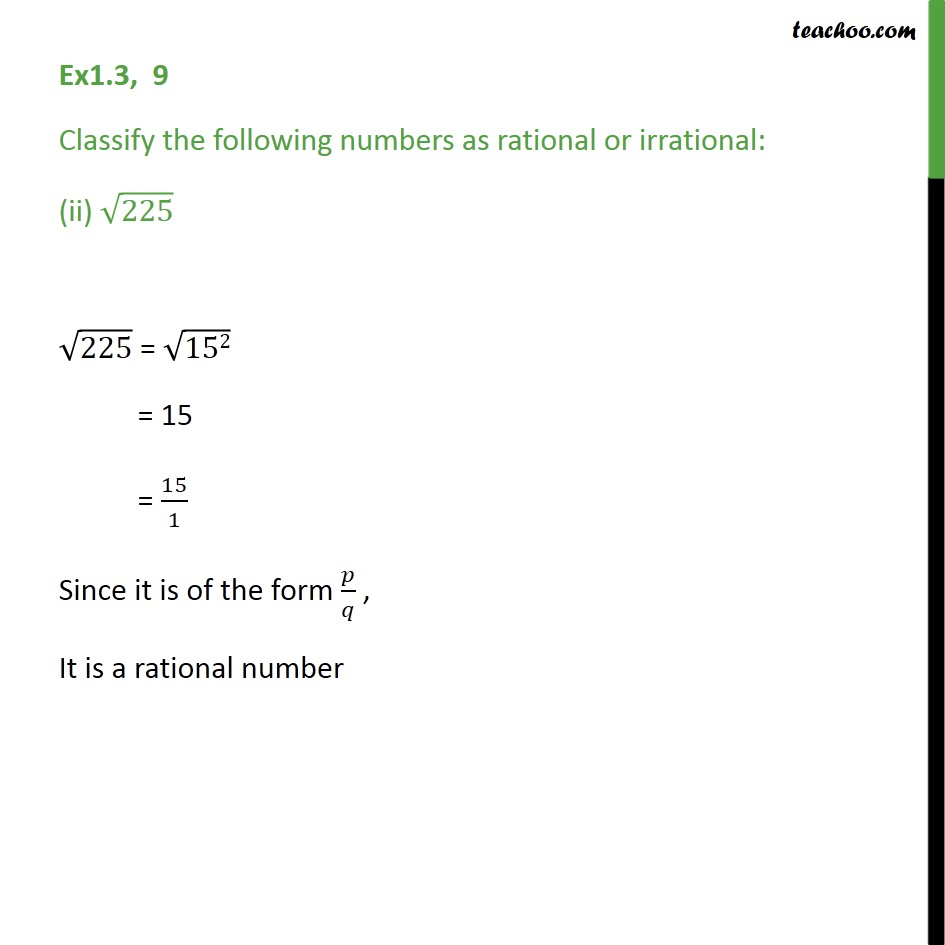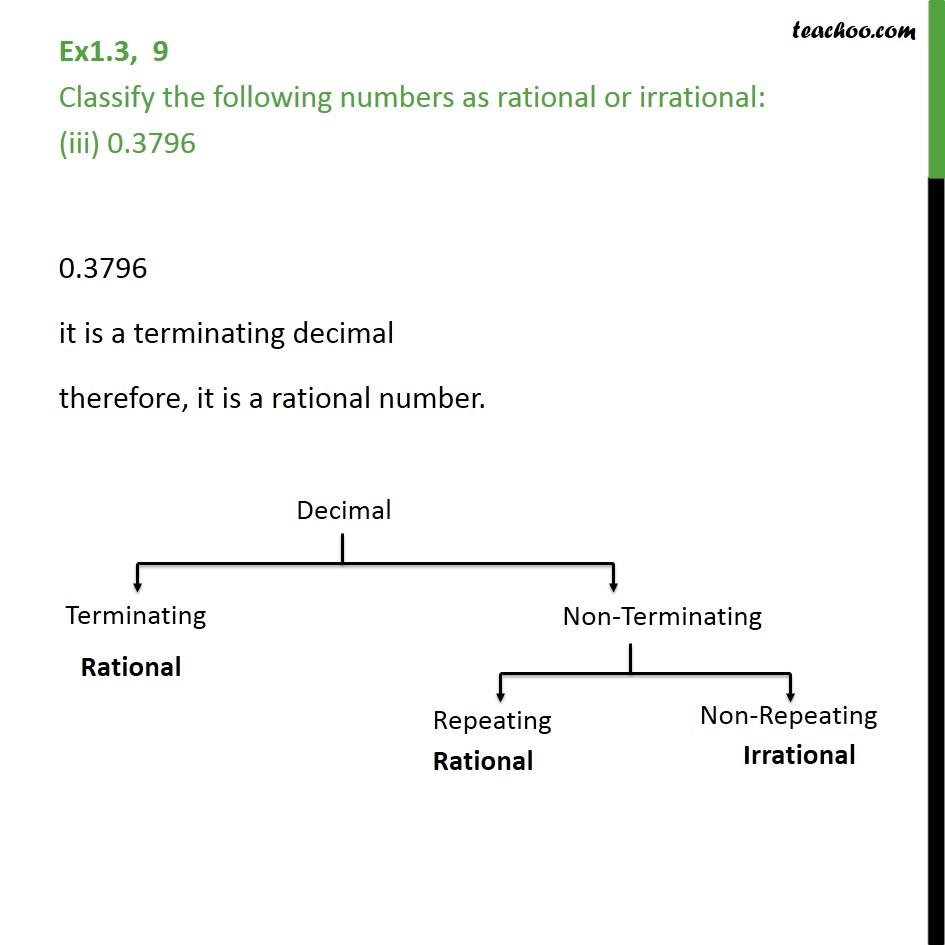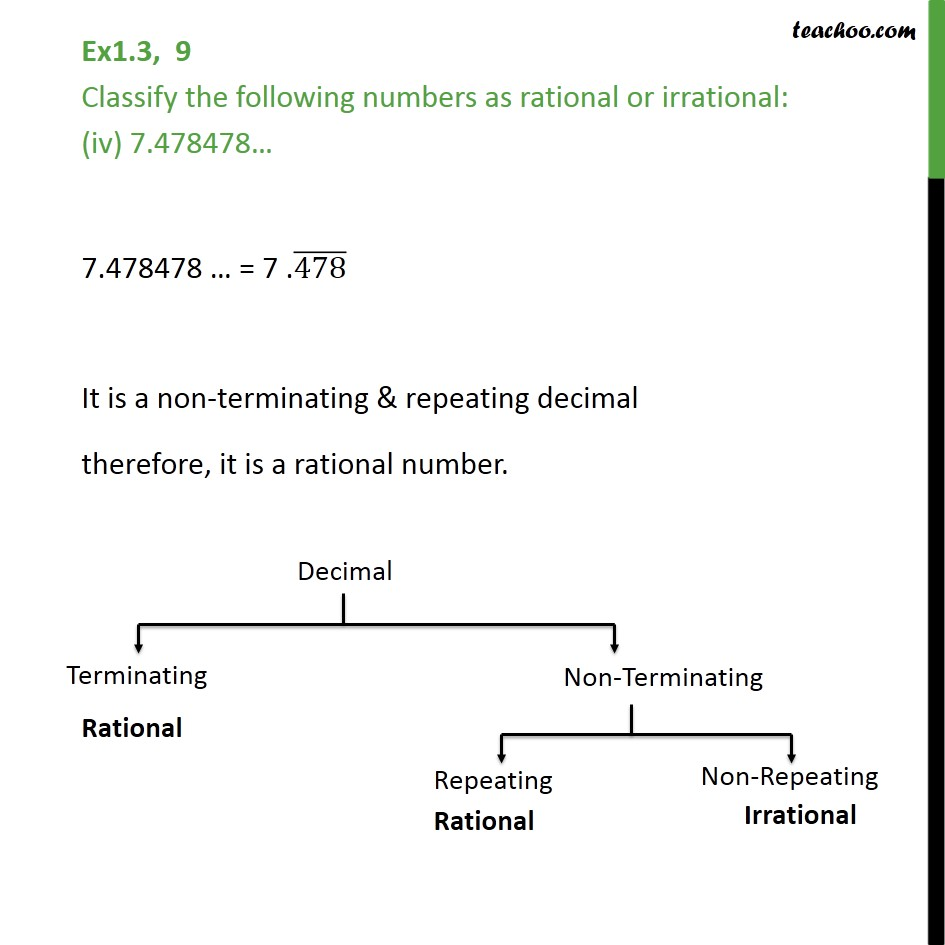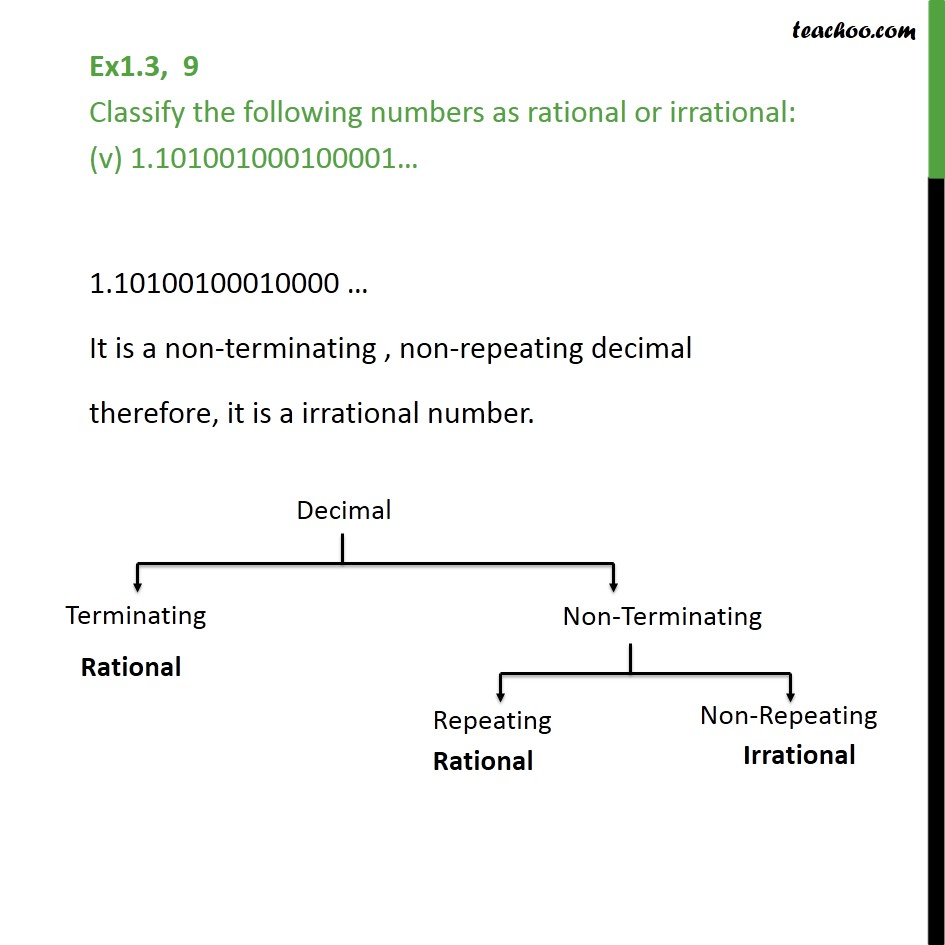1. Chapter 1 Class 9 Number Systems
2. Serial order wise
3. Ex 1.3

Transcript

Ex1.3, 9 Classify the following numbers as rational or irrational: (i) 23 We cant write 23 as square of some number So 23 is not it a perfect square, Hence ,it is an irrational number. Ex1.3, 9 Classify the following numbers as rational or irrational: (ii) 225 225 = 152 = 15 = 15/1 Since it is of the form / , It is a rational number Ex1.3, 9 Classify the following numbers as rational or irrational: (iii) 0.3796 0.3796 it is a terminating decimal therefore, it is a rational number. Ex1.3, 9 Classify the following numbers as rational or irrational: (iv) 7.478478 7.478478 = 7 .(478) It is a non-terminating & repeating decimal therefore, it is a rational number. Ex1.3, 9 Classify the following numbers as rational or irrational: (v) 1.101001000100001 1.10100100010000 It is a non-terminating , non-repeating decimal therefore, it is a irrational number.

Ex 1.3